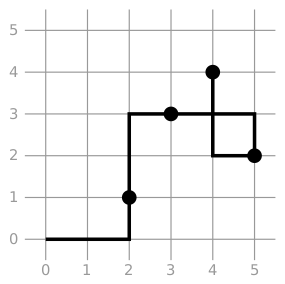# #536. 【IOI2019】折线

• 该折线从 $(0,0)$ 开始（即 $sx_0=sy_0=0$ ）。
• 该折线经过所有的小圆点（它们不必是线段的端点）。
• 该折线仅包括水平线段和竖直线段（对于构成该折线的连续两个点，其 $x$ 坐标或 $y$ 坐标相等，且不重合）。
• 折线中的线段可以相交或重叠；换言之，平面上的每个点可以被任意数量的线段覆盖。

### 输出格式

• $-2\times 10^9 \le sx_i,sy_i \le 2 \times 10^9$。

### 样例一

#### input

4
2 1
3 3
4 4
5 2

#### output

2 0
2 3
5 3
5 2
4 2
4 4

#### explanation### 数据范围

#### 计分方式

• $i$ 分，如果 $c_i=t,(1 \le i \le 10)$。
• $i+\frac{c_i-t}{c_{i+1}-c_i}$ 分，如果 $c_{i+1} < t < c_i(1 \le i \le 9)$。
• $0$ 分，如果 $t > c_1$。
• $10$ 分，如果 $t < c_{10}$。